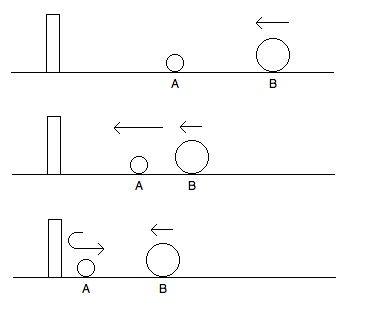# Computing π with Billiards (Mathematical Puzzles/Riddles, Part IV)

In honor of this past Monday being Pi day, I thought I would post a special mathematical oddity/riddle related to calculating π. Many of you may be familiar with the famous Buffon’s needle problem, in which π is estimated by throwing needles on the floor and using some elementary calculus and probability theory. Here we will describe another amusing method for computing π (perhaps not very practical), involving some basic physics!

COMPUTING PI VIA BILLIARDSSpecial thanks to Y. Fan, who communicated the following problem.

Let’s consider two billiard balls of equal mass constrained to move along the real axis. Suppose that Ball A is initially stationary and is located at the point x = 1 on the real axis, while Ball B is traveling towards it from the right in with some fixed velocity. Suppose moreover that there is a wall at x = 0 off of which the billiard balls reflect. We will of course assume that there is no friction, and that the billiard balls are point masses which are perfectly elastic.

In this simple situation, it is evident that there will be a total of three collisions amongst the two billiard balls and the wall. First, B strikes A, resulting in A moving to the left (with the same velocity as B originally had), and B becoming stationary. Then A reflects off the wall, reversing its velocity. Finally, A strikes B from the left, resulting in A becoming stationary and B moving off to the right. In particular, note that the number of collisions is independent of the masses of the billiard balls (given that they are equal), and the incoming velocity of B.

Now suppose that the balls are not of equal mass, but rather that B is significantly more massive than A, say 100 times more massive. Then after B initially strikes A, A will move rapidly to the left, while B will still be moving to the left with a slightly diminished velocity. As before, A then reflects off of the wall and strikes B, which slows down B slightly. After enough cycles of A reflecting off the wall and striking B, B will start to move to the right, and it is easily seen that eventually B will be moving to the right with sufficient speed so that no more collisions of any kind occur. In order that we do not have to worry about B itself getting too close to the wall, let us pretend that every so often we pick up the wall and move it to the left. (This does not affect the velocities of the balls.)

We can now ask the question: in total, how many collisions occur amongst the two billiard balls and the wall? It turns out that if B is 100 times as massive as A, there are (perhaps up to plus or minus one extra collision), 31 total collisions (regardless of the incoming velocity of B and the numerical values of the masses of A and B). If B is 10000 times as massive, it turns out that there are approximately 314 collisions! We thus have the following:

Prove that if B is 102n times as massive as A, then the total number of collisions within our system of billiard balls is approximately π times 10n.

No advanced physics is needed to solve this problem – you can get by with only the conservation of energy and the conservation of momentum!

HINTS FOR THE BILLIARDS PROBLEM

Breaking with tradition a bit, I thought I would post a hint for the billiards problem, since it’s a bit more computational than the previous problems I have posted. There aren’t any other riddles in this post, so if you don’t want to read the hint, you can turn off your computer now and go frolic in the sun. Even given the hint, there’s still some work, but it may be helpful for getting and overall idea of the solution:

Let’s represent the velocity of A (vA) and the velocity of B (vB) at any given time as a point (vAvB) in the plane. Because of the conservation of energy, throughout the evolution of our system this point is constrained to lie on some ellipse. Under a judicious change of coordinates, we can turn this ellipse into a circle. Can you relate the point (vAvB) before a collision to the point(vAvB) after a collision? Are they related in a geometric way? Can you phrase the point at which no more collisions occur as a statement about where(vAvB) lies on the circle?